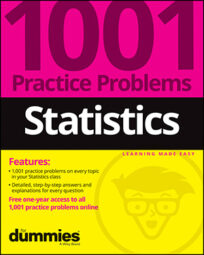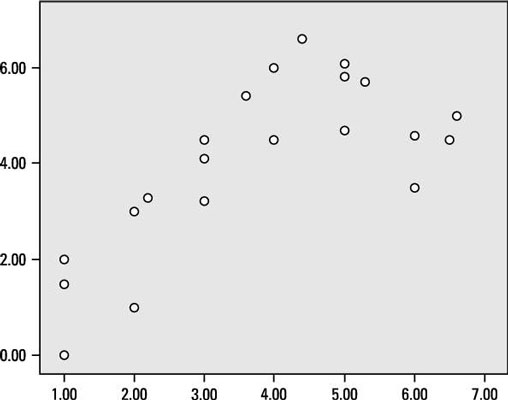##### Statistics: 1001 Practice Problems For Dummies (+ Free Online Practice)With simple linear regression, you look for a certain type of relationship between two quantitative (numerical) variables (like high-school GPA and college GPA.) This special relationship is a linear relationship — one whose pairs of data resemble a straight line.

In this scatter plot, the two variables plotted are quantitative (numerical). The correlation is r = 0.75.Credit: Illustration by Ryan Sneed

## Sample questions

1. In looking at this scatter plot, which of the following violations of a necessary condition for fitting a regression line is observed?

A. The variables aren't numeric.

B. Their correlation isn't strong enough.

C. Their relationship isn't linear.

D. Choices (B) and (C)

E. None of the above.

Answer: C. Their relationship isn't linear.

The variables are numeric, and a correlation of 0.75 is sufficient to perform linear regression. However, the relationship of the points in the scatter plot isn't linear. The points initially have a positive relationship but then curve downward into a negative relationship.

2. The equation for calculating the least-squares regression line is y = mx + b. If two variables have a negative relationship, which letter is guaranteed to be negative?